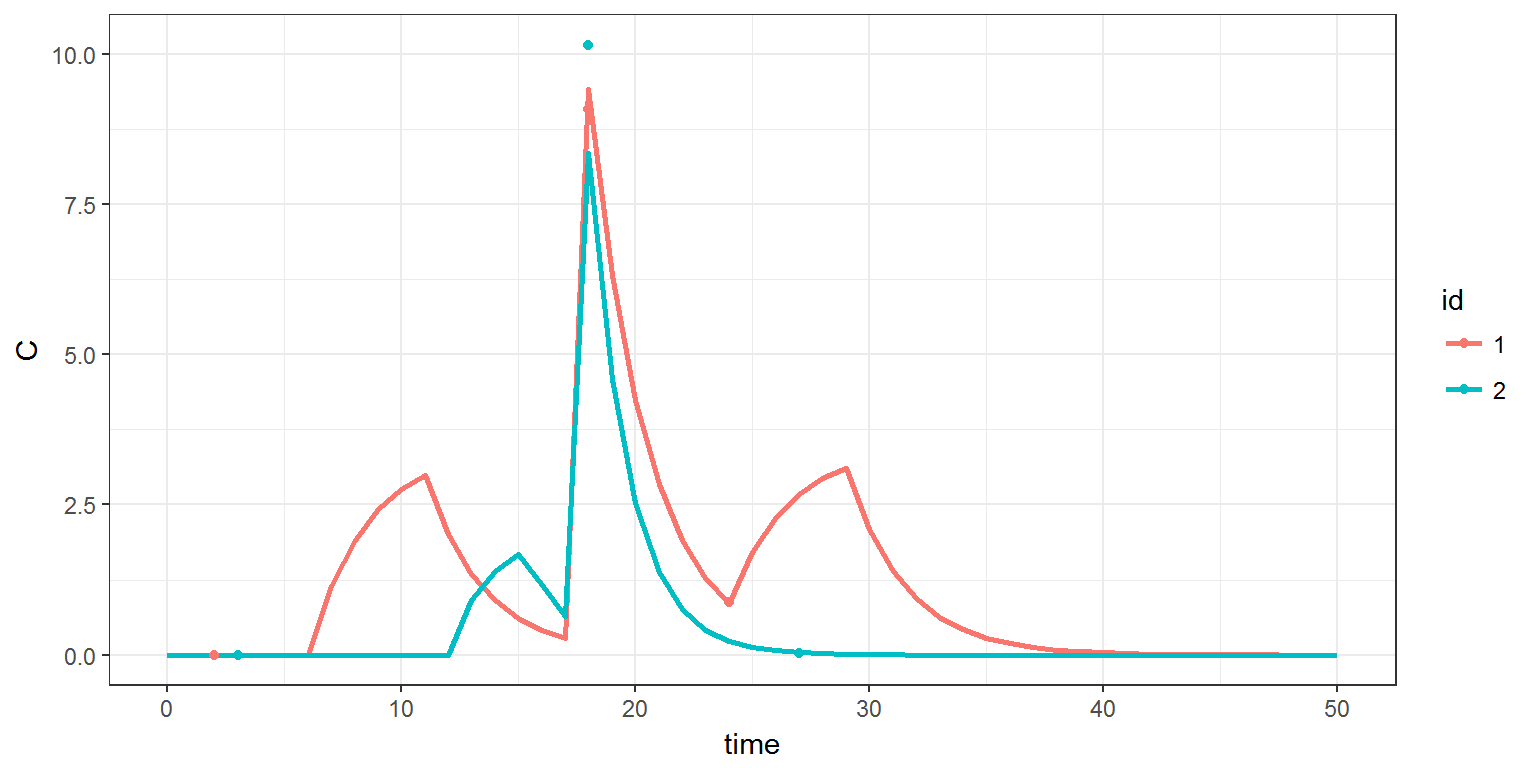R scripts: data.R

Mlxtran codes: model/groupI.txt ; model/groupII1.txt ; model/groupII2.txt

$$\newcommand{\esp}{\mathbb{E}\left(#1\right)} \newcommand{\var}{\mbox{Var}\left(#1\right)} \newcommand{\deriv}{\dot{#1}(t)} \newcommand{\prob}{ \mathbb{P}\!(#1)} \newcommand{\eqdef}{\mathop{=}\limits^{\mathrm{def}}} \newcommand{\by}{\boldsymbol{y}} \newcommand{\bc}{\boldsymbol{c}} \newcommand{\bpsi}{\boldsymbol{\psi}} \def\pmacro{\texttt{p}} \def\like{{\cal L}} \def\llike{{\cal LL}} \def\logit{{\rm logit}} \def\probit{{\rm probit}} \def\one{{\rm 1\!I}} \def\iid{\mathop{\sim}_{\rm i.i.d.}} \def\simh0{\mathop{\sim}_{H_0}} \def\df{\texttt{df}} \def\res{e} \def\xomega{x} \newcommand{\argmin}{{\rm arg}\min_{#1}} \newcommand{\argmax}{{\rm arg}\max_{#1}} \newcommand{\Rset}{\mbox{\mathbb{R}}} \def\param{\theta} \def\setparam{\Theta} \def\xnew{x_{\rm new}} \def\fnew{f_{\rm new}} \def\ynew{y_{\rm new}} \def\nnew{n_{\rm new}} \def\enew{e_{\rm new}} \def\Xnew{X_{\rm new}} \def\hfnew{\widehat{\fnew}} \def\degree{m} \def\nbeta{d} \newcommand{\limite}{\mathop{\longrightarrow}\limits_{#1}} \def\ka{k{a}} \def\ska{k{\scriptscriptstyle a}} \def\kel{k{e}} \def\skel{k{\scriptscriptstyle e}} \def\cl{C{\small l}} \def\Tlag{T\hspace{-0.1em}{lag}} \def\sTlag{T\hspace{-0.07em}{\scriptscriptstyle lag}} \def\Tk{T\hspace{-0.1em}{k0}} \def\sTk{T\hspace{-0.07em}{\scriptscriptstyle k0}} \def\thalf{t{1/2}} \newcommand{\Dphi}{\partial_\pphi #1} \def\asigma{a} \def\pphi{\psi} \newcommand{\stheta}{{\theta^\star}} \newcommand{\htheta}{{\widehat{\theta}}}$$

# 1 Introduction

Individual parameters and individual designs (dosage regimens and observation times) can be defined in external data files, or as inline’’ data frames.

Function inlineDataFrame is extremly useful for converting formatted text into data frames.

# 2 Individual parameters defined in a data frame

Let us start with the PK model for iv administration implemented in groupI.txt:

[LONGITUDINAL]
input = {V, k}
EQUATION:
C = pkmodel(V,k)


Suppose that we want to use this model for computing the predicted concentration of 4 individuals with different PK parameters stored in the file data/data1.txt.

 id     V      k
1    12   0.15
2     9   0.25
3     8   0.15
4    11   0.20


Column named id (taking the values 1, 2, 3, 4) is required in order to identify these 4 individuals. The names of the two other columns should match with the names of the parameters of the model (i.e. V and k).

We can then load this table and use it as input for simulx:

p = read.table("data/data1.txt",header=TRUE)

C <- list(name="C", time=seq(0, 10, by=1))

res1a <- simulx(model     = "model/groupI.txt",
parameter = p,
output    = C,

print(ggplot(data=res1a$C, aes(x=time, y=C, colour=id)) + geom_line(size=1))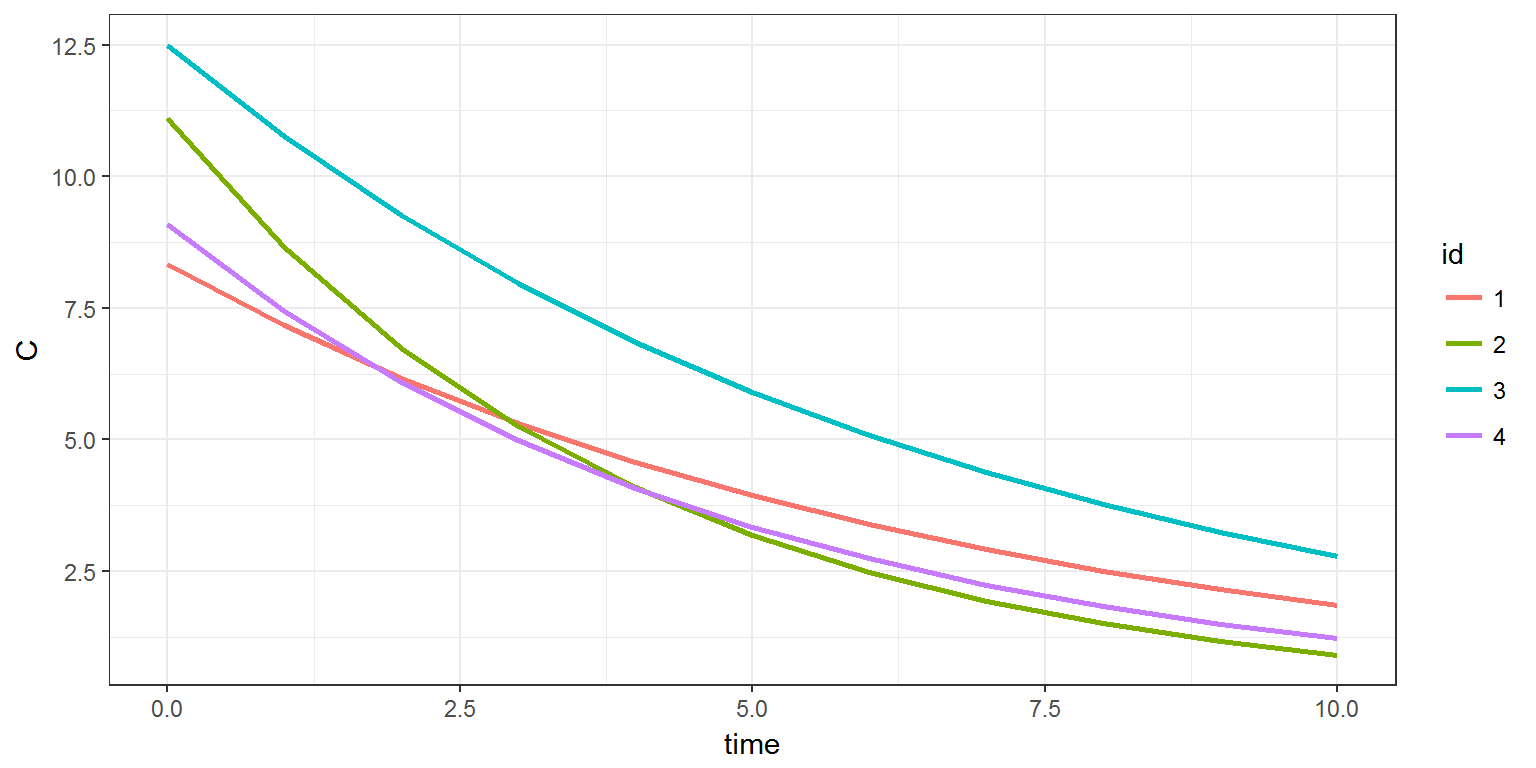Instead of reading the parameter values in an external data file, this data can be provided directly in the R script using the function inlineDataFrame which converts formatted text into a data frame: p <- inlineDataFrame(" id V k 1 12 0.15 2 9 0.25 3 8 0.15 4 11 0.20 ") print(p) ## id V k ## 1 1 12 0.15 ## 2 2 9 0.25 ## 3 3 8 0.15 ## 4 4 11 0.20 Assume now that the four individuals share some parameters ($$k$$ in this example). It is then possible to define the input argument parameter as a list which combines a data frame (for the parameters which are different) and a vector (for the parameters which are shared) V <- inlineDataFrame(" id V 1 12 2 9 3 8 4 11 ") k <- c(k=0.2) p <- list(V, k) res1b <- simulx(model = "model/groupI.txt", parameter = p, output = C, treatment = adm) print(ggplot(data=res1b$C, aes(x=time, y=C, colour=id)) + geom_line(size=1))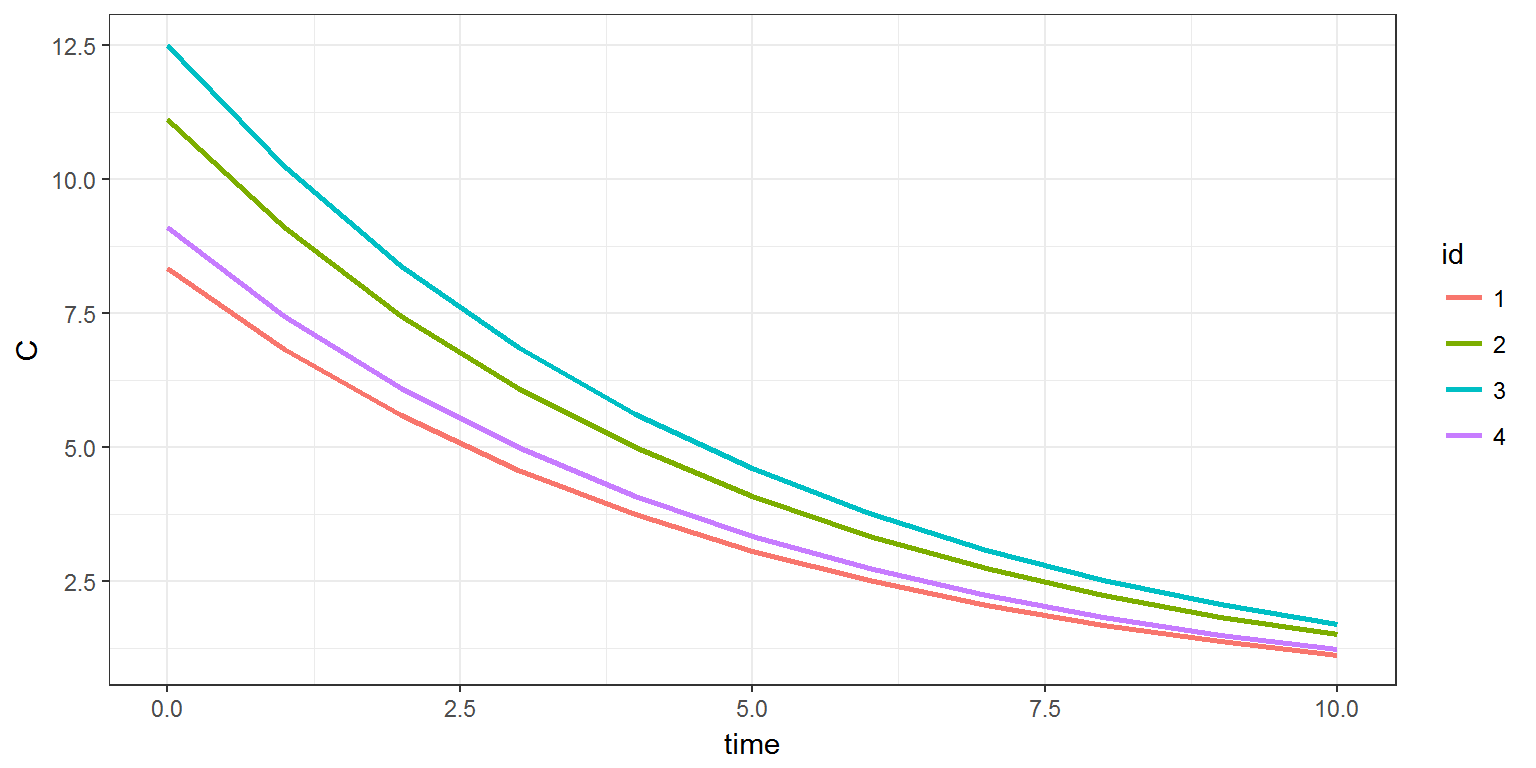# 3 Treatment defined in a data frame

Individual dosage regimens can also be defined in an external data file, or in an inline’’ data frame:

adm <- inlineDataFrame("
id  time  amount  rate
1      1    100   0
1     12     50   10
1     24    100   10
2      6     75   15
2     18    100   0
2     24     75   15
")

p   <- c(V=10, k=0.15)
C  <- list(name="C", time=seq(0, 50, by=1))

res2a <- simulx(model     = "model/groupI.txt",
parameter = p,
output    = C,

print(ggplot(data=res2a$C, aes(x=time, y=C, colour=id)) + geom_line(size=1))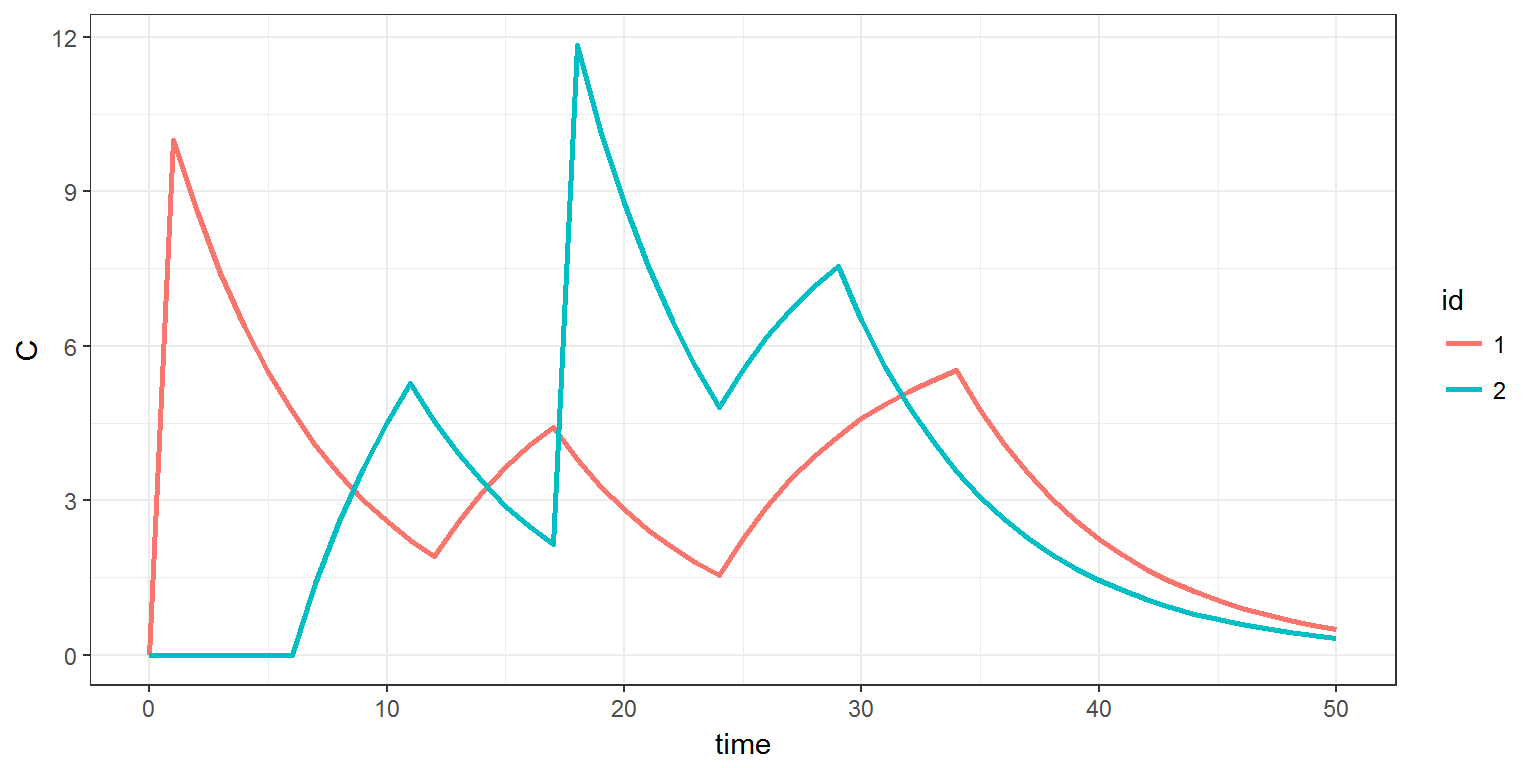It is possible to combine these individual dose regimens with doses defined for all the patients:. in this example, 2 additional doses of 50mg are given to all the patients: res2b <- simulx(model = "model/groupI.txt", parameter = p, output = C, treatment = list(adm, list(time=c(20,40),amount=50)), settings = list(load.design=TRUE)) print(ggplot(data=res2b$C, aes(x=time, y=C, colour=id)) + geom_line(size=1))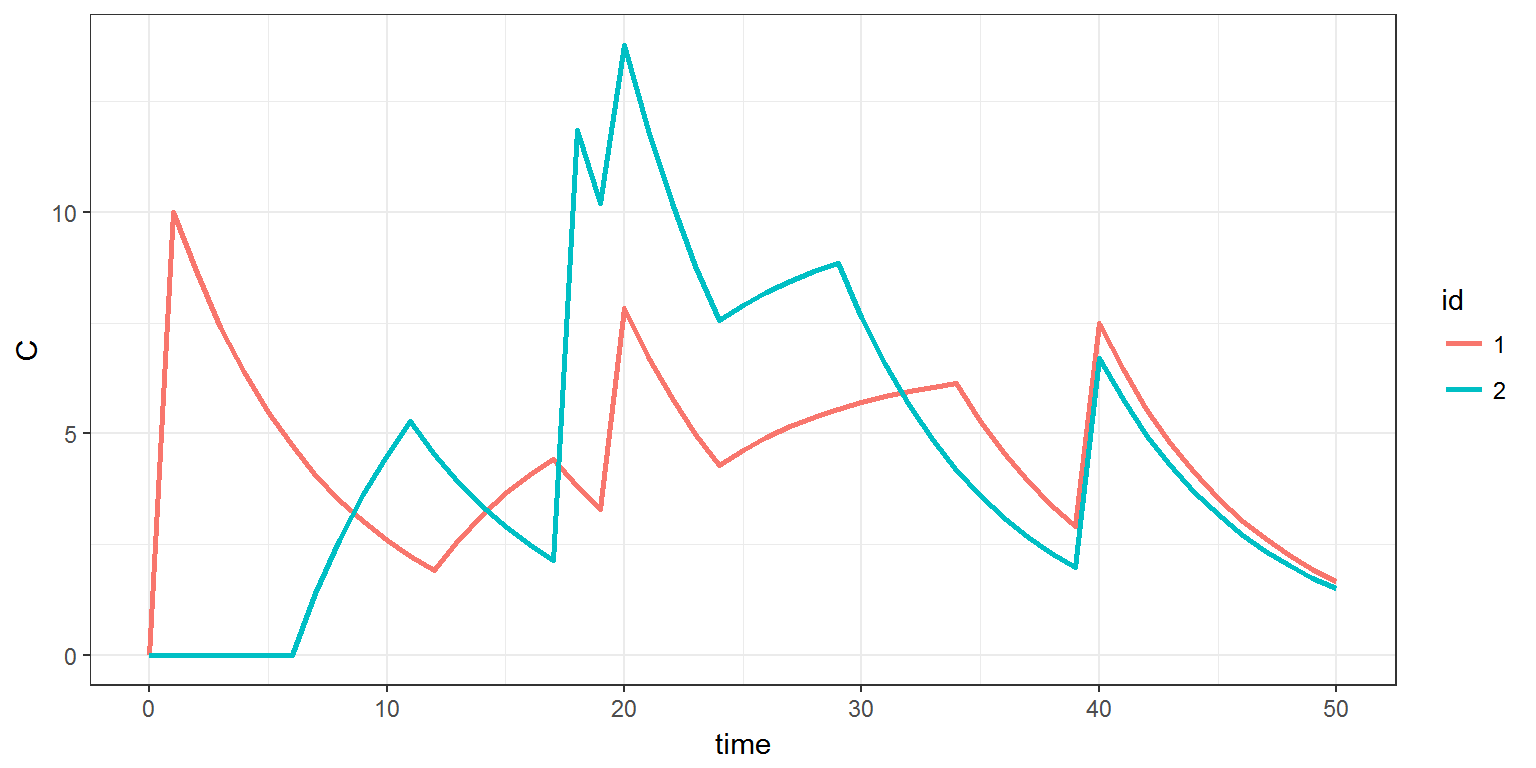# 4 Observation times defined in a data frame

We will use in this example the model implemented in groupII1.txt where observed concentrations are defined.

[LONGITUDINAL]
input = {V, k, a}
EQUATION:
C = pkmodel(V,k)
DEFINITION:
y = {distribution=lognormal, prediction=C, sd=a}


Different individual can have different observation designs, i.e. different observation times $$(t_{ij})$$. Individual observation designs can be defined in a data file, or in an inline data frame:

p   <- c(V=10, k=0.15, a=0.2)

design.y  <- inlineDataFrame("
id  time
1     3
1     6
1     9
1    12
2     2
2    10
2    18
")
y  <- list(name="y",  time=design.y)

res3 <- simulx(model     = "model/groupII1.txt",
parameter = p,
output    = y,

geom_point(data=res4$y, aes(x=time, y=y, colour=id)))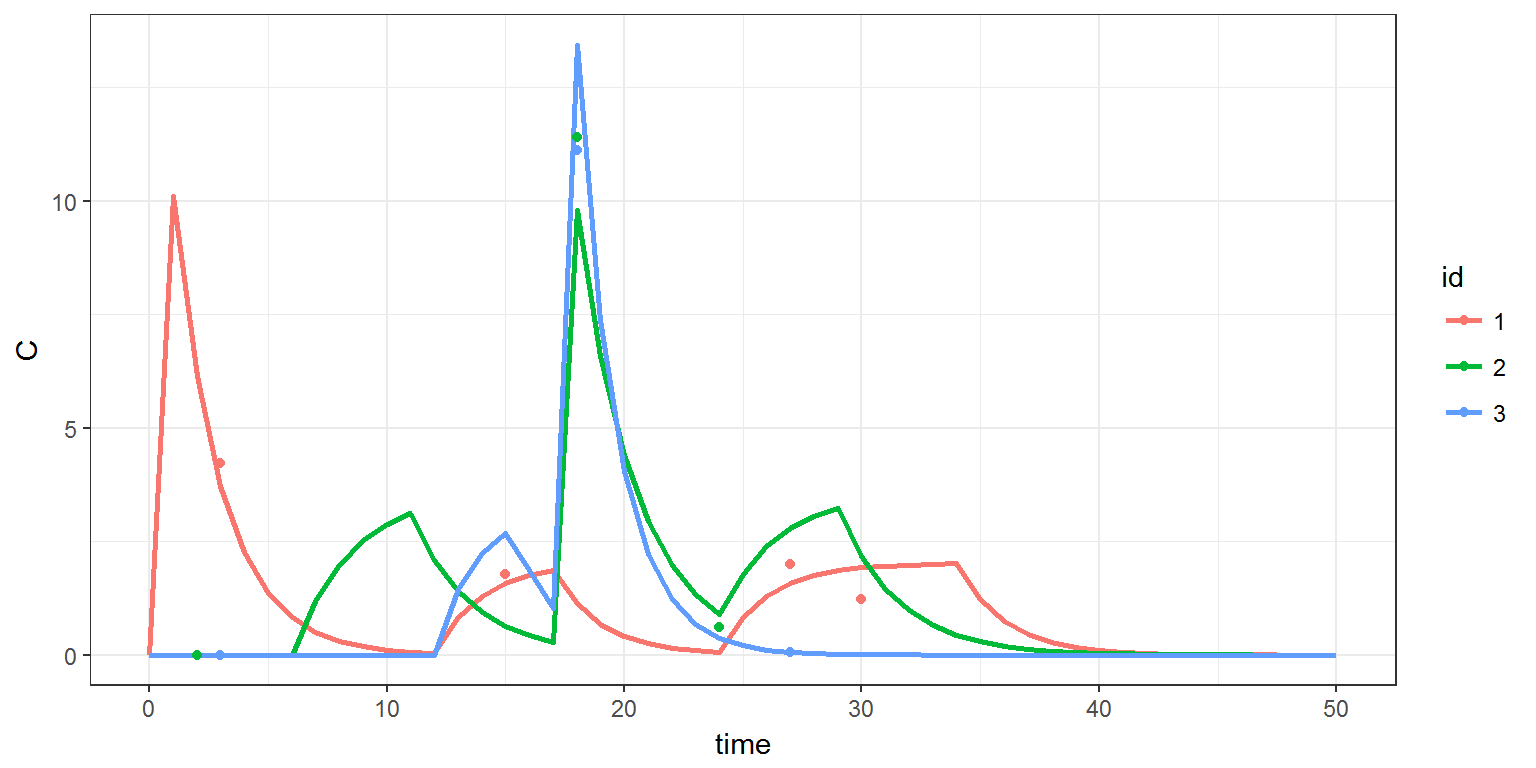# 6 Sampling/resampling individuals from a database We may want to use the same individual information provided by these 3 individuals, but for a different number of subjects. in this example, we create 8 individuals by resampling the 3 original individuals: res5a <- simulx(model = "model/groupII2.txt", parameter = list(p.pop,p.indiv), output = list(out.C, out.y), treatment = adm, group = list(size=8)) print(ggplot() + geom_line(data=res5a$C, aes(x=time,y=C,colour=id),size=1) +
geom_point(data=res5a$y, aes(x=time, y=y, colour=id)))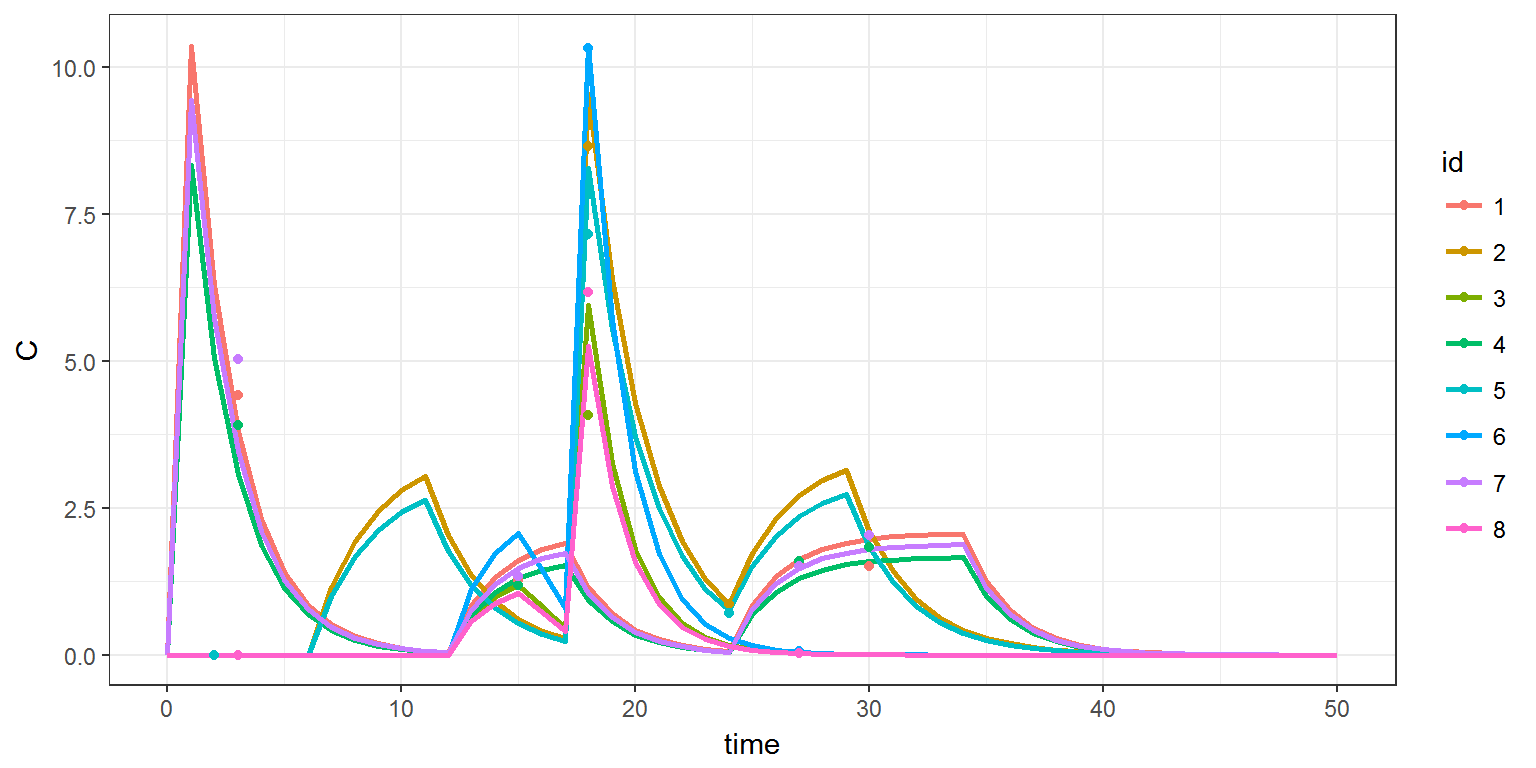You should note that the level of randomization () is not defined when subjects are resampled. By default, settings$replacement=F, which means that the new id’s are sampled within the original ones without replacement

print(res5a$originalId) ## newId oriId ## 1 1 1 ## 2 2 2 ## 3 3 3 ## 4 4 1 ## 5 5 2 ## 6 6 3 ## 7 7 1 ## 8 8 3 New id’s are sampled with replacement by setting settings$replacement=T

res5b <- simulx(model     = "model/groupII2.txt",
parameter = list(p.pop,p.indiv),
output    = list(out.C, out.y),
print(res5b$originalId) ## newId oriId ## 1 1 1 ## 2 2 1 ## 3 3 2 ## 4 4 2 ## 5 5 3 ## 6 6 2 ## 7 7 2 ## 8 8 3 The same method can be used for generating less individuals than in the original data base: res5c <- simulx(model = "model/groupII2.txt", parameter = list(p.pop,p.indiv), output = list(out.C, out.y), treatment = adm, group = list(size=2)) print(res5c$originalId)
##   newId oriId
## 2     2     3
print(ggplot() + geom_line(data=res5c$C, aes(x=time,y=C,colour=id),size=1) + geom_point(data=res5c$y, aes(x=time, y=y, colour=id)))